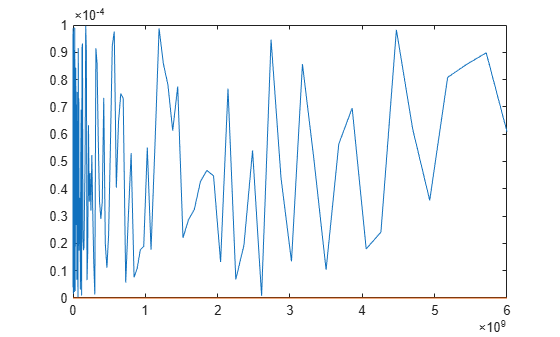# rational

Perform rational fitting to complex frequency-dependent data

## Description

Use the `rational` object and an interpolative algorithm to create a rational fit to frequency-dependent data.

The complex frequencies are given by the equation:

## Creation

### Syntax

``fit = rational(freq,data)``
``fit = rational(s)``
``fit = rational(___,tol)``
``[fit,error] = rational(___)``
``fit = rational(___,Name,Value)``

### Description

````fit = rational(freq,data)` returns a rational object with complex frequencies using the given frequency vector and network parameter data.```

example

````fit = rational(s)` returns a rational object for N-port S-parameters.```
````fit = rational(___,tol)` returns a rational object `fit` that satisfies a relative error tolerance. Specify `tol` after any of the input argument combinations from the previous syntaxes. ```
````[fit,error] = rational(___)` also returns the error of the fit. Use any of input argument combinations from the previous syntaxes.```

example

````fit = rational(___,Name,Value)` sets properties using one or more name-value arguments. For example, `fit = rational(s,'Tolerance',-34)`sets the relative error tolerance in decibels for the `fit`. Specify name-value arguments after any of the input arguments from the previous syntaxes.```

### Input Arguments

expand all

Nonnegative frequencies, specified as a vector of nonnegative frequencies in Hz.

Data Types: `double`

Network parameter data, specified as a vector, a 2-D array or a 3-D array. The length of the data values must equal the length of the frequency values.

Relative error tolerance, specified as a scalar less than or equal to zero. `tol` value sets the input for the `'Tolerance'` property.

Data Types: `double`

N-port S-parameters, specified as an N-by-N matrix of elements of S sharing identical poles.

## Properties

expand all

Relative error tolerance, specified as a scalar less than or equal to zero.

Data Types: `double`

Behavior of fit for large S-parameters, specified as `true` or `false`. When `true`, the direct term in the fit is set to zero so that the rational fit `F(S)` tends to zero as S approaches infinity. When `false`, a nonzero direct term is allowed.

Data Types: `logical`

Maximum number of poles, specified as a scalar nonnegative integer.

Data Types: `double`

Error metrics in `rational` object, specified as one of the following:

• If you specify `'ErrorMetric'` as `'default'`, the `rational` object distributes the error evenly.

• If you specify `'ErrorMetric'` as `'Relative'`, the `rational` object fits both peaks and valleys or gets smaller error for smaller values.

Data Types: `char`

Ignores low-level noise in data, specified as a scalar.

Example: `NoiseFloor = -60`

Data Types: `double`

Pole stability, specified as a logical `true` (`1`) or `false` (`0`). When you specify true, all the poles of the fit are stable. When you specify false,the poles can be anywhere in the complex plane.

Example: `Casual = false`

Maximum value of the quality factor of the poles of the fit, specified as a positive scalar.

Example: `Qlimit = 1100`

Data Types: `double`

Data reduction, specified as a logical `1` (`true`) or `0` (`false`). When you specify true, the function reduces the data for the fitter to save memory and computation time. Reduce data for the fitter in order to save memory and computation time, specified as a logical `true` or `false`.

Example: `ColumnReduce = false`

Display options for the fitting algorithm of the rational object, specified as one of the following:

• `'off'`— No display

• `'on'`— Printed information

• `'plot'`— Plots of the interpolation progress

• `'both'`— Both printed information and plots.

Data Types: `char`

## Object Functions

 `timeresp` Time response for rational object and `rationalfit` function object `stepresp` Step-signal response for rational object and `rationalfit` function object `freqresp` Frequency response of rational object and `rationalfit` function object `pwlresp` Calculate time response of piecewise linear input signal `impulse` Impulse response for rational function object `ispassive` Return true if `rationalfit` output is passive at all frequencies `makepassive` Enforce passivity of `rationalfit` output or a rational object `passivity` Plot passivity of N-by-N `rationalfit` function output `generateSPICE` Generate SPICE file from `rationalfit` of S-parameters

## Examples

collapse all

Create S-Parameters from the file named `passive.s2p`.

`S = sparameters('passive.s2p');`

Perform rational fitting of the S-parameters.

`fit = rational(S);`

Create an S-Parameters object from the file named `default.s2p`. Perform rational fitting of the S-Parameters.

```S = sparameters('default.s2p'); fit = rational(S,'Display', 'plot')```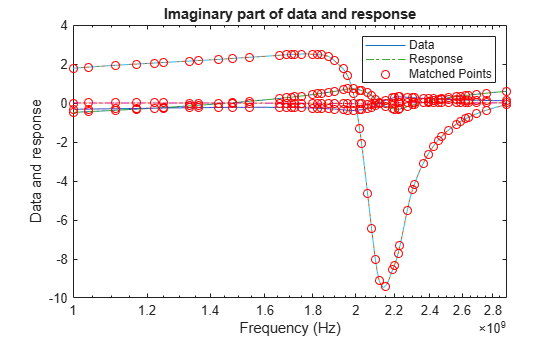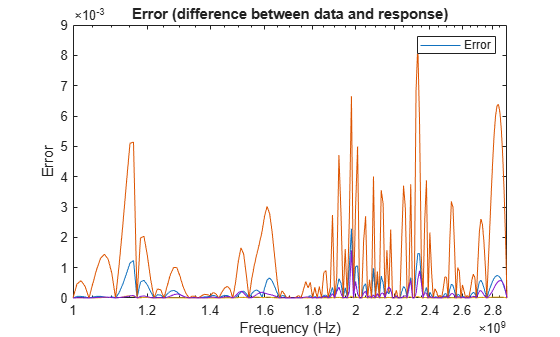```fit = rational with properties: NumPorts: 2 NumPoles: 44 Poles: [44x1 double] Residues: [2x2x44 double] DirectTerm: [2x2 double] ErrDB: -22.6464 ```

Calculate the zeros, poles, gain, and DC gain of the rational object.

`[z,p,k,dcgain] = zpk(fit)`
```z=2×2 cell array {43x1 double} {43x1 double} {43x1 double} {43x1 double} ```
```p=2×2 cell array {44x1 double} {44x1 double} {44x1 double} {44x1 double} ```
```k = 2×2 109 × -2.5743 -0.0556 -0.6980 -1.3221 ```
```dcgain = 2×2 0.0933 -0.0302 -1.1079 0.4746 ```

Create an S-parameters object from the specified S2P file.

```S = sparameters('sawfilterpassive.s2p'); f = S.Frequencies;```

Create a `rational` object with the tolerance of `-40` dB.

`fit = rational(S,-40);`

Compare the fit to the data. You can see the deviations at the smaller values on a semi-log plot.

```dresp = freqresp(fit,f); plot(f,20*log10(abs(squeeze(S.Parameters(2,1,:)))),... f,20*log10(abs(squeeze(dresp(2,1,:)))),f,20*log10(abs(squeeze(S.Parameters(2,1,:)-dresp(2,1,:))))) title('Default Fitting for sawfilterpassive.s2p'); ylabel('dB'); xlabel('Frequency (Hz)'); legend('Data','Fit','Error','Location','northwest');```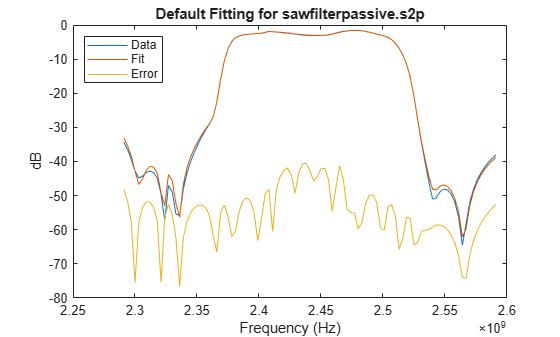Create a `rational` object with `'ErrorMetric'` set to `'Relative'` to fit both peaks and valleys.

`rfit = rational(S,-40,'ErrorMetric','Relative');`

Compare the fit to the data. The peaks and valleys are fitted.

```rresp = freqresp(rfit,f); figure(2) plot(f,20*log10(abs(squeeze(S.Parameters(2,1,:)))),... f,20*log10(abs(squeeze(rresp(2,1,:)))), f, 20*log10(abs(squeeze(S.Parameters(2,1,:)-rresp(2,1,:))))); title('Relative Error Fitting for sawfilterpassive.s2p'); ylabel('dB'); xlabel('Frequency (Hz)'); legend('Data','Fit','Error','Location','northwest');```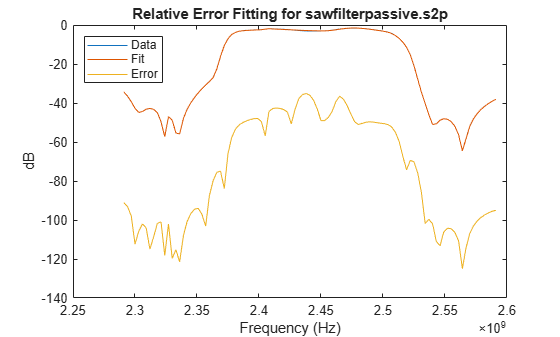Create an S-parameters object from the specified S2P file.

```S = sparameters('passive.s2p'); f = S.Frequencies; data = S.Parameters;```

Set one of the data entries to zero.

`data(2,2,:) = 0;`

Create a `rational` object with the tolerance of `-40` dB.

`fit = rational(f,data,-40);`

Compare the fit to the data. The fit and the data match closely.

```xresp = freqresp(fit,f); figure(3) plot(f,abs(squeeze(data(2,1,:))),f,abs(squeeze(xresp(2,1,:))))```Add noise to the data and create a `rational` object.

```rng(1); noisyData = data + 1e-4 * rand(size(S.Parameters)); nfit = rational(f,noisyData,-40);```

Compare the fit to the data with noise. Noise cannot be fitted because this is a data with a higher order fit with a worse error metric.

```nresp = freqresp(nfit,f); figure(4) plot(f,abs(squeeze(noisyData(2,2,:))),f,abs(squeeze(nresp(2,2,:))))```Create a `rational` object with the noise floor of `-60` dB and plot the fit. The fitter ignores low-level noise.

```ffit = rational(f,noisyData,-40,'NoiseFloor',-60); fresp = freqresp(ffit,f); figure(5) plot(f,abs(squeeze(noisyData(2,2,:))),f,abs(squeeze(fresp(2,2,:))))```